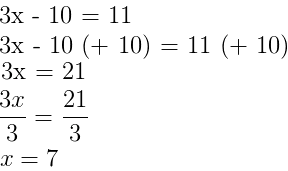# 2 1.1 Understanding Equations and Basic Math

Mathematics can sometimes feel like a different language. With enough practice, you can become fluent in the language of numbers. Let’s review the concept of ‘equation’. An equation says that two ‘things’ are equal with the use of an equal sign ‘=’. For example:

x + 2 = 6

That equation says: what is on the left (x+2) is equal to what is on the right (6) of the equal sign. This can be a powerful tool as we aim to understand human movement with data (numbers) collected. The equations may get a bit more complicated but the rules remain the same.

A formula is a ‘rule’ that use mathematical symbols. It usually consists of an equal sign and two or more variables. For example. the formula for force (as you will see later in the course) is:

F = ma

This can be stated as: the force acting on an object is equal to the mass of the object multiplied by the acceleration of the object. It can be convenient with formulas to have basic algebra skills. In algebra, the goal is to get the letter or symbol (also called the unknown) on one side of the equation (usually the left) and the numbers on the other side.

The golden rule of algebra is: anything you do on one side of the equation, you must do on the other side. For example, if you want to add 10 from one side of the equation, you must add 10 from the other.In cases where the variable shows up twice in the equation, you should try to get all of the variables on one side of the equation and all of the numbers on the other:

x + 23 = 3x +45

 1. Initial Equation / Problem x + 23 = 3x + 45 2. Subtract x from each side x – x + 23 = 3x – x + 45 Result 23 = 2x + 45 3. Subtract 45 from each side 23 – 45 = 2x + 45 – 45 Result -22 = 2x 4. Divide both sides by 2 -22 = 2x Result x = -11

To become proficient in algebra, you should practice.

### A note on the symbols used in this textbook

You’ll notice that we used letters to symbolize a variable. For example, the value for ‘force’ will feature as ‘F’ in an equation. Although some symbols are universal (‘m’ for mass and ‘a’ for acceleration), some are not. Physics, engineering and biomechanics may use different symbols for the same thing (both p and L can be used for momentum). Sometimes, the same symbol can have different meanings in the same field. For example, a capital W can be used for both ‘weight’ and ‘work’. You’ll have to take great care in understanding the meaning of each variable based on the context they are presented in.

A symbol can have up to four parts: the main variable, a leading superscript, a following superscript and a following subscript:

xp’1

The main variable (p in the example above) represents the variable you are quantifying. The leading superscript (x) let’s the reader know the frame of reference. X represents the horizontal axis and y the vertical axis. We’ll discuss this in detail later in the book. The following superscript is important if you are keeping track of the variable over time. Time zero does not have a superscript, time at point 1 has a single prime, time at point 2 has a double prime and so on… The following subscript is important if you are quantifying the variable for more than one body. If you have two runners and you are reporting both their momentum, person 1 would have a moment p1 and person 2, p2.